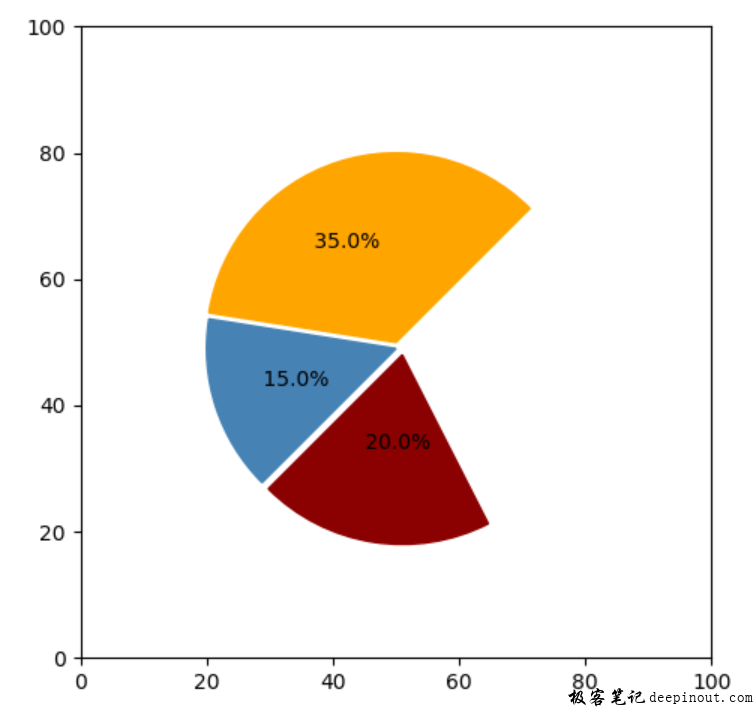# Matplotlib 使用模块patches的Wedge实现饼图

Matplotlib 使用patches绘制几何图形学习了patches模块，也知道了一些简单使用，但是知识要在应用中才发现它的好处。本文就来演示Wedge的使用，使用它来实现饼图。饼图最合适用来表示百分比了，因为一个圆就表示百分之百，某一部分就可以使用扇形来表示，这样看起来就比较明了，占得多的面积的就是百分比大的。当然，也可以直接使用matplotlib里的饼图来实现，但是这里主要是演示Wedge模块。当你需自定义一些数据图时，就可以采用类似的方法来实现，也就是实现自定义图表，这样灵活性更大。如果你掌握了这种方法，应付各种实验的论文，各种课堂演示的稿件，都会得心应手，真正做到图文并茂。sample = [350, 150, 200, 300]

total = sum(sample)

per = [i/float(total) for i in sample]

angles = [360*i for i in per]


wedge1 = mpatches.Wedge((50, 50), 30, delta, delta + sum(angles[0:1]), color = 'orange')

host.text(36, 65, f"{per* 100 : 3.1f}%")


import numpy as np
import matplotlib.pyplot as plt
import matplotlib.patches as mpatches

#

fig, host = plt.subplots()  #创建子图

host.grid(False)

host.set_aspect("equal")

host.set_xlim(0, 100)

host.set_ylim(0, 100)

#

sample = [350, 150, 200, 300]

total = sum(sample)

per = [i/float(total) for i in sample]

angles = [360*i for i in per]

delta = 45

wedge1 = mpatches.Wedge((50, 50), 30, delta, delta + sum(angles[0:1]), color = 'orange')

host.text(36, 65, f"{per* 100 : 3.1f}%")

#

wedge2 = mpatches.Wedge((50, 49), 30, delta + sum(angles[0:1]), delta + sum(angles[0:2]), color = 'steelblue')

host.text(28, 43, f"{per* 100 : 3.1f}%")

#

wedge3 = mpatches.Wedge((51, 48), 30, delta + sum(angles[0:2]), delta + sum(angles[0:3]), color = 'darkred')

host.text(44, 33, f"{per* 100 : 3.1f}%")

plt.tight_layout()

plt.show()


• 回顶